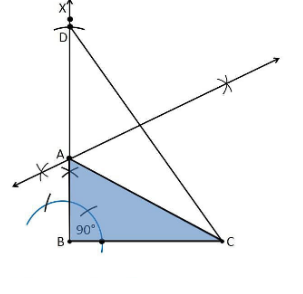# Construct a right triangle whose base is 12cm and sum of its hypotenuse and other side is 18 cm.Procedure

1. Draw a line segment of base BC = 12 cm

2. Measure and draw ∠B = 90° and draw the ray BX

3. Using a compass measure AB+AC = 18 cm.

4. Considering B as a centre and draw an arc at the point be D on the ray BX

5. Join DC

6. Now draw the perpendicular bisector of the line CD and the intersection point is taken as A.

7. Now join AC

8. Hence, ABC is the required triangle.